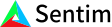1. Bob Anderson
×
IntuitiveML

## What is a confusion matrix? How does it work? Why do we care?

Jun 26, 2020

A confusion matrix is a nice way of visualizing the performance of your models. In my video last week on accuracy, precision and recall, I made a mistake while drawing my confusion matrix. Thanks to Reddit users u/MlecznyHotS, u/Alouis07, u/dafeviizohyaeraaqua, and u/wasperen for pointing it out. Let’s fix my mistake.

Last week, when drawing the confusion matrix, I mixed up all the locations of where true positives, true negatives, false positives, and false negatives go on the confusion matrix. In reality, a confusion matrix looks like this – but how do you read it? There are four boxes that correspond to the number of examples where your predicted value matches the actual value, or not, depending on the square. The top row is predicting positive and the bottom row is predicting negative. Similarly, the first column is what is actually positive, and the second column is what is actually negative. So to understand what the top right box means, it’s in the predicted positive row with actually negative column – it’s the number of examples where your model guessed positive when in reality it was negative – the number of false positives. Quickly going through the rest of the boxes – the top left and bottom right are simple - top left is where you predicted positive and it was positive I.e., top left is the true positive square, and bottom right is where you predicted negative and it was negative so that’s the true negative square. Finally, the bottom left is where you predicted negative but it was actually a positive, so that’s the false negative square.

Confusion matrices can be confusing to read sometimes for two reasons. One, a lot of tables will just label the axes as actual and predicted instead of labeling the values – while identical in function, I find that the prior takes up less space but the latter is way easier to read. The second reason that confusion matrices can be confusing is because people will sometimes flip the axes – the x axis will become predicted instead of actual and the y axis will become actual instead of predicted. Even on Wikipedia (article linked in the description), all of the examples have rows represent different actual classes but for the last non-example confusion matrix, that is, the table that defines very clearly what is a true positive, what is a false positive etcetera, that confusion matrix uses rows to represent different predicted classes instead! So you just have to be careful and watch your axis when you are reading someone’s results.

Why do we care about confusion matrices? Why do we care about the individual values of true positives, false positives, false negatives, and true negatives? Because different problems care more about certain values than others. Let’s say that you are responsible for developing a model that does drug testing. After initial development, you see you are 99% accurate! Yay!

Actual Positive Actual Negative
Predicted Positive 100 400
Predicted Negative 100 59400

However, if you look at the confusion matrix, you see that in reality, you have 4 times as many false positives as true positives. That means that if every person who takes your test and gets a positive result gets thrown in jail for drug use, 4 for every 5 people in jail or 80% of people who use your test are actually innocent. So you have to carefully consider your evaluation metrics and always double check the confusion matrix for weird or interesting results that your model outputs.